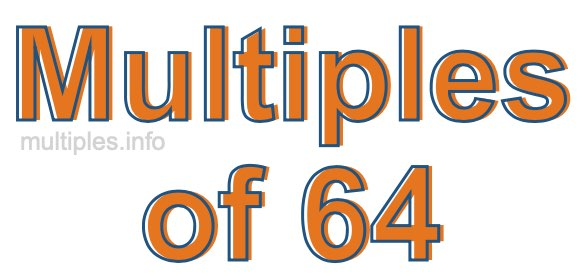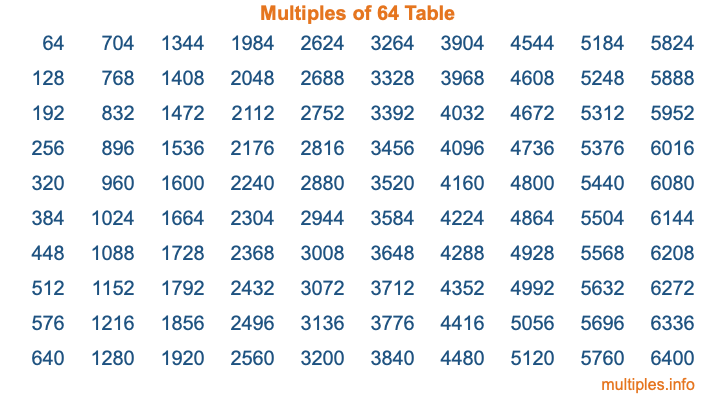Multiples of 64Welcome to the Multiples of 64 page. Here we will first teach you everything you will ever need to know about the multiples of 64, and then give you a study guide summary of everything we taught you to make sure you remember it all. Use this page to look up facts and learn information about the multiples of 64. This page will make you a multiples of sixty-four expert!

Definition of Multiples of 64
Multiples of 64 are all the numbers that when divided by 64 equal an integer. Each of the multiples of 64 are called a multiple. A multiple of 64 is created by multiplying 64 by an integer.

Therefore, to create a list of multiples of 64, you start with 1 multiplied by 64, then 2 multiplied by 64, then 3 multiplied by 64, and so on for as long as you want. Thus, the list of the first five multiples of 64 is 64, 128, 192, 256, and 320. To see a larger list of multiples of 64, see the printable image of Multiples of 64 further down on this page. We also have a category where you can choose any nth multiple of 64.

Multiples of 64 Checker
The Multiples of 64 Checker below checks to see if any number of your choice is a multiple of 64. In other words, it checks to see if there is any number (integer) that when multiplied by 64 will equal your number. To do that, we divide your number by 64. If the the quotient is an integer, then your number is a multiple of 64.

Is  a multiple of 64?

Least Common Multiple of 64 and ...
A Least Common Multiple (LCM) is the lowest multiple that two or more numbers have in common. This is also called the smallest common multiple or lowest common multiple and is useful to know when you are adding our subtracting fractions. Enter one or more numbers below (64 is already entered) to find the LCM.

Check out our LCM Calculator if you need more details about the Least Common Multiple or if you need the LCM for different numbers for adding and subtraction fractions.

nth Multiple of 64
As we stated above, 64 is the first multiple of 64, 128 is the second multiple of 64, 192 is the third multiple of 64, and so on. Enter a number below to find the nth multiple of 64.

th multiple of 64

Multiples of 64 vs Factors of 64
64 is a multiple of 64 and a factor of 64, but that is where the similarities end. All postive multiples of 64 are 64 or greater than 64. All positive factors of 64 are 64 or less than 64.

Below is the beginning list of multiples of 64 and the factors of 64 so you can compare:

Multiples of 64: 64, 128, 192, 256, 320, etc.

Factors of 64: 1, 2, 4, 8, 16, 32, 64

As you can see, the multiples of 64 are all the numbers that you can divide by 64 to get a whole number. The factors of 64, on the other hand, are all the whole numbers that you can multiply by another whole number to get 64.

It's also interesting to note that if a number (x) is a factor of 64, then 64 will also be a multiple of that number (x).

Multiples of 64 vs Divisors of 64
The divisors of 64 are all the integers that 64 can be divided by evenly. Below is a list of the divisors of 64.

Divisors of 64: 1, 2, 4, 8, 16, 32, 64

The interesting thing to note here is that if you take any multiple of 64 and divide it by a divisor of 64, you will see that the quotient is an integer.

Multiples of 64 Table
Below is an image of the first 100 multiples of 64 in a table. The table is in chronological order, column by column. The first column has the first ten multiples of 64, the second column has the next ten multiples of 64, and so on.The Multiples of 64 Table is also referred to as the 64 Times Table or Times Table of 64. You are welcome to print out our table for your studies.

Negative Multiples of 64
Although not often discussed or needed in math, it is worth mentioning that you can make a list of negative multiples of 64 by multiplying 64 by -1, then by -2, then by -3, and so on, to get the following list of negative multiples of 64:

-64, -128, -192, -256, -320, etc.

Multiples of 64 Summary
Below is a summary of important Multiples of 64 facts that we have discussed on this page. To retain the knowledge on this page, we recommend that you read through the summary and explain to yourself or a study partner why they hold true.

There are an infinite number of multiples of 64.

A multiple of 64 divided by 64 will equal a whole number.

64 divided by a factor of 64 equals a divisor of 64.

The nth multiple of 64 is n times 64.

The largest factor of 64 is equal to the first positive multiple of 64.

64 is a multiple of every factor of 64.

64 is a multiple of 64.

A multiple of 64 divided by a divisor of 64 equals an integer.

64 divided by a divisor of 64 equals a factor of 64.

Any integer times 64 will equal a multiple of 64.

Multiples of a Number
Here you can get the multiples of another number, all with the same attention to detail as we did for multiples of 64 on this page.

Multiples of
Multiples of 65
Did you find our page about multiples of sixty-four educational? Do you want more knowledge? Check out the multiples of the next number on our list!